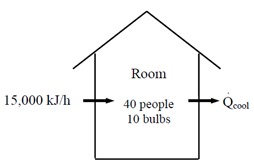## Installing AC Units

A classroom that normally contains 40 people is to be air-conditioned with window AC units that have a 5-kW cooling capacity. A person at rest may be assumed to dissipate heat at a rate of 360 kJ/h. There are 10 lightbulbs in the room, each with a rating of 100 W. The rate of heat transfer to the classroom through the walls and the windows is estimated to be 15,000 kJ/h. If the room air is to be maintained at a constant temperature of 21°C, determine the number of window AC units required.Hint
Assuming there is no other heat dissipating equipment like computers, TVs, etc in the room, the total cooling load is determined from:
$$\dot{Q}_{cooling}=\dot{Q}_{lights}+\dot{Q}_{people}+\dot{Q}_{heat\:gain}$$$Hint 2 The number of air-conditioning units needed is: $$\frac{\dot{Q}_{cooling}}{cooling\:capacity}$$$
Assuming there is no other heat dissipating equipment like computers, TVs, etc in the room, the total cooling load is determined from:
$$\dot{Q}_{cooling}=\dot{Q}_{lights}+\dot{Q}_{people}+\dot{Q}_{heat\:gain}$$$where $$\dot{Q}_{lights}=10\times 100\:W=1\:kW$$$
$$\dot{Q}_{people}=40\times 360\:kJ/h=4\:kW$$$$$\dot{Q}_{heat\:gain}=15,000\:kJ/h=4.17\:kW$$$
Substituting,
$$\dot{Q}_{cooling}=1+4+4.17=9.17\:kW$$$Thus, the number of air-conditioning units needed is: $$\frac{9.17\:kW}{5\:kW/unit}=1.83\rightarrow 2\:units$$$
2 units